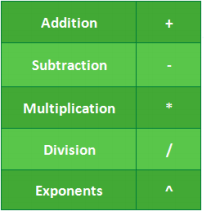## How To Use Google Sheets To Make Report Cards

It is the beginning of a new session. As innovative educators it is best to look out for ways to make learning more fun and teaching more effective. One of the ways we can improve on our content delivery is to incorporate technology in our teaching process. Technological tools can make your life as a teacher simpler and more impactful.

Spreadsheet programs like Microsoft Excel and OpenOffice.org Calc can be used to manage grade sheets effectively. Not only do they provide a safe backup compared to paper sheets, they are also provide ways to keep grade records.

They can also be used to automate complicated tasks from calculating averages to providing detailed statistical analyses of the class’s performance. This guide deals with the basic most common tasks, here are the ways you can start using Google sheets to enhance your productivity.

Using Common Formulas

Google sheets has a bunch of built-in formulas for carrying out large statistical and data manipulation tasks. You can also combine formulas to create more powerful calculations and strings tasks together.

To use add a formula to a cell, begin typing with a = sign in a cell followed by the formula name. Google sheets will auto-fill or suggest formulas based on your first letters, so you don’t need to remember every formula. The basic formulas can help you make quick calculations such as sum, average, count on the data you have for your classroom.Using Basics Statistical Data

As earlier stated, one way to use Microsoft excel as an educator is for reporting student academic performance. At the end of a semester, you will want to calculate a total point value and a percentage score for each student. You can program the spreadsheet to do the work on the values in appropriate cells.

Basic statistical data can show you things you would otherwise take days sorting out in paper form. Some of the important statistic you can do with excel are:

• Average: Find the average of a range of cells
• Min: Find the lowest value in a range of cells (=SUM \$1: \$20)
• Max: Find the highest value in a range of cells (=SUM \$1: \$20)
• Count: Count the values in a range of cells

Using Round Off and Percentages

In creating a Grade work Book, you have the opportunity to work with real test scores, which might have variations in decimals. How do you make quick decisions by rounding up these variations into decimals.  Test whether the formulas you have learnt can work for you. Enter the names of students and scores of real assignments and use the round up & percentage formulas to:

• Create percentage scores for each student
• Roundup scores & use minimum & maximum to calculate highest & lowest performing studentsSource: exceljet.net

Creating a report sheet template

At the end of a session, you will want to calculate a total point value, and probably a percentage for each student. To do this you can program the spread sheet to do the work on the values in appropriate cells.  If you specify what grade should cover a particular range (say Grade A will be for 75 – 100), then Google Sheet will do the work by identifying each grade scores and aligning a Grade score next to it!

The Nest IF Solution

=IF(Score>=75,”A”,IF(Score>=60,”B”,IF(Score>=50,”C”,IF(Score>=40,”D”,”F”))))As seen above this sample report above, using the simple formulas, we were able to add T1, T2, T3,T4,T5 representing 5 test scores of this class. Next we calculated the percentage scores, in the PCNT column, next, we used the round off formula to round up the numbers, then we used the Nest If Solution to calculate the grade of each student, finally, we were able to the highest performing student using the maximum score formula.